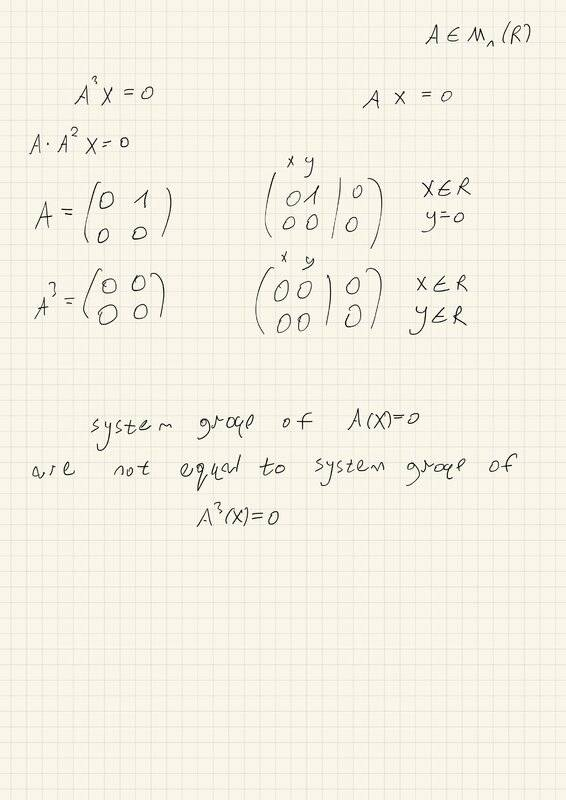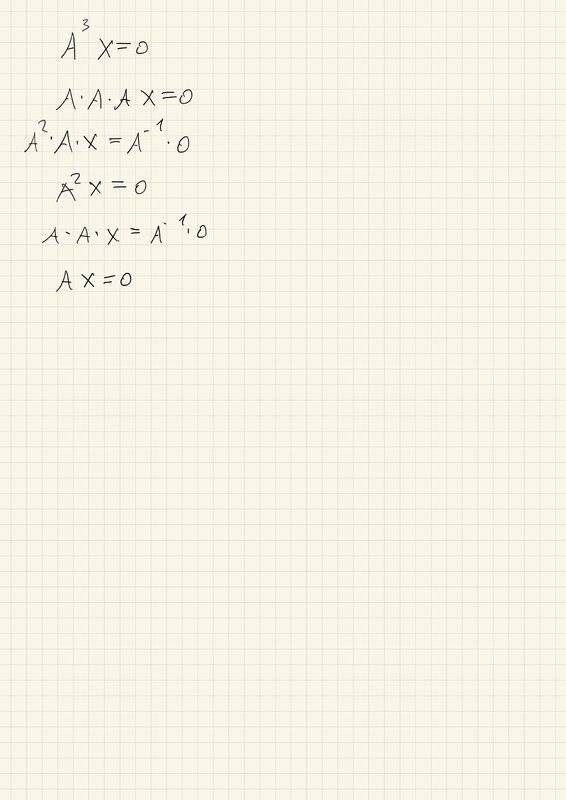# Help with solution group of a Homogeneous system

• afik
But I don't know that, I just computed it.Do you know how to calculate it then?Yes, I can calculate it.In summary, the solution group of the system A^3X=0 is not equal to the solution group of the system AX=0. If this is true you will prove it, if not give a counterexample.

#### afik

Summary:: need help with solution group of Homogeneous system

Is the solution group of the system A^3X = 0
, Is equal to the solution group of the system AX = 0

If this is true you will prove it, if not give a counterexample.

thank you.

Have you tried anything?

I tried but I failed..
The matrix A ∈ Mn (R)

Well, you can show us your work, it could be a good point to startsecond attempt:The second attempt has the problem that ##A^{-1}## doesn't exist for ##A \in M_n(\mathbb{R})##.

Gaussian97 said:

The second attempt has the problem that ##A^{-1}## doesn't exist for ##A \in M_n(\mathbb{R})##.
I do not know if I do it right in the first attempt...
Why in the second attempt A^-1 does not exist?

"Is the solution group of the system ##A^3 x = 0## equal to the solution group of the system ##Ax = 0##?"

And you literally wrote:
"system group of ##A x = 0 ## are not equal to system group of ##A^3 x = 0##"

For the ##A^{-1}## question is easy, the example you use, let ##A = \begin{pmatrix}0&1\\0&0\end{pmatrix}## then
$$A \in M_2(\mathbb{R}), \qquad \nexists A^{-1}$$

Gaussian97 said:
"Is the solution group of the system ##A^3 x = 0## equal to the solution group of the system ##Ax = 0##?"

And you literally wrote:
"system group of ##A x = 0 ## are not equal to system group of ##A^3 x = 0##"

For the ##A^{-1}## question is easy, the example you use, let ##A = \begin{pmatrix}0&1\\0&0\end{pmatrix}## then
$$A \in M_2(\mathbb{R}), \qquad \nexists A^{-1}$$
I wrote:
"system group of ##A x = 0 ## are not equal to system group of ##A^3 x = 0##"

I wrote it down, but I do not know if my counter-argument is true there, or if I am wrong.

Ah well, then that's a completely different thing. What in that argument do you think can be wrong?

Gaussian97 said:
Ah well, then that's a completely different thing. What in that argument do you think can be wrong?
If I know what can be wrong I finish the homework... I don't know :(

Ok, so what things in your argument are you absolutely sure are correct?

If Ax= 0 then certainly A^3x=A^2(Ax)= A^2(0)= 0 for A any linear operator. So the solution set of A is a subset of the solution set of A^3. The question is "are there x that satisfy A^3x= 0 but not Ax= 0?

Can you help me more, I need it for tomorrow :(

Yes, that's correct. But in your prove you essentially did 3 things:
1. Calculate the solutions of ##Ax=0##
2. Calculate the matrix ##A^3##
3. Calculate the solutions of ##A^3 x = 0##

What of these steps do you think may be wrong? And what of these steps you are 100% sure are right?

I think that 1 and 3 are correct and 2 is wrong.

Well, so let's take a closer look at the computation of ##A^3##, do you have the intermediate steps?

Gaussian97 said:
Well, so let's take a closer look at the computation of ##A^3##, do you have the intermediate steps?
No, I don't have any idea.

Have you studied how matrices are multiplied? If you know that to compute ##A^3## is just computing first
$$A^2 = A \cdot A$$
and then
$$A^3 = A \cdot A^2$$